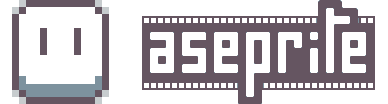# Trying to calculate Image for entire group

I’m writing a script that I would like to iterate through all the layers on the root level of my sprite and for each one that has a name that starts with the _ symbol, it should export it as an image. I’m doing this to speed up creating a menu with buttons on it so I can keep everything in a single file and let the script export the different pieces.

This was working fine when everything was an image layer, but I’m having trouble now that I’m trying to export groups. Ie, if I have a group that starts with an _ with several images in it, I’d like to export the combined image for the entire group. The Image:drawImage() command don’t work because it overwrites alpha instead of blending it. My custom blit command works, but it’s slow. Is there a way to calculate the combined image for everything within a group?

``````local spr = app.activeSprite
if not spr then
return print('No active sprite')
end

local path, title = spr.filename:match("^(.+[/\\])(.-).([^.]*)\$")
local rgba = app.pixelColor.rgba

function image_bounds(layer)
local rect = nil
if layer.isGroup then
for i, child in ipairs(layer.layers) do
if rect == nil then
rect = image_bounds(child)
else
rect:union(image_bounds(child))
end
end
return rect
else
local cel = layer:cel(1)
local img = cel.image:clone()
return cel.bounds
end
end

function blit(imgSrc, imgDest, position)
local w = imgSrc.width
for j = 0, imgSrc.height - 1, 1 do
local destY = j + position.y
if destY >= 0 and destY < imgDest.height then

for i = 0, imgSrc.width - 1, 1 do
local destX = i + position.x
if destX >= 0 and destX < imgDest.width then
local pSrc = imgSrc:getPixel(i, j)
local pDest = imgDest:getPixel(destX, destY)
local cs = Color(pSrc)
local cd = Color(pDest)

local f = cs.alpha / 255.0
local rr = cs.red * f + cd.red * (1 - f)
local gg = cs.green * f + cd.green * (1 - f)
local bb = cs.blue * f + cd.blue * (1 - f)
local aa = cs.alpha + cd.alpha

rr = math.min(math.max(rr, 0), 255)
gg = math.min(math.max(gg, 0), 255)
bb = math.min(math.max(bb, 0), 255)
aa = math.min(math.max(aa, 0), 255)

imgDest:drawPixel(destX, destY, rgba(rr, gg, bb, aa))

end
end
end
end
end

function draw_layer(layer, destImage, destBounds)
if layer.isGroup then
for i, child in ipairs(layer.layers) do
draw_layer(child, destImage, destBounds)
end
else
local cel = layer:cel(1)
local img = cel.image:clone()
local bounds = cel.bounds
blit(img, destImage, Point(bounds.x - destBounds.x, bounds.y - destBounds.y))
--        destImage:drawImage(img, Point(bounds.x - destBounds.x, bounds.y - destBounds.y))
end
end

function build_image(layer)
local bounds = image_bounds(layer)
local image = Image(bounds.width, bounds.height)

app.alert("bounds " .. bounds.x .. " " .. bounds.y .. " " .. bounds.width .. " " .. bounds.height )

draw_layer(layer, image, bounds)
return image
end

for i, layer in ipairs(spr.layers) do
layer_name = layer.name:match("^_(.*)\$")
if layer_name then

local img = build_image(layer)
local save_path = path .. title .. "_" .. layer_name .. ".png"
img:saveAs(save_path)

--        if layer.isTransparent then
--layer.isGroup
--layer.layers

-- if layer.isGroup then

-- local cel = layer:cel(1)
-- local img = cel.image:clone()
-- local save_path = path .. title .. "_" .. layer_name .. ".png"